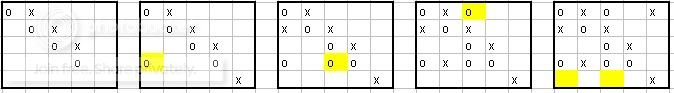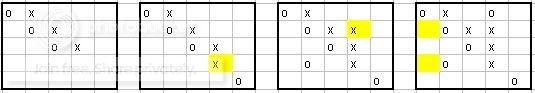# A Transversal Achievement Game on a Grid

Two players alternately write O's (first player) and X's (second player) in the unoccupied cells of an n x n grid. The first player (if any) to occupy some transversal (i.e., a set of n cells having no two cells in the same row or column) is the winner. What is the outcome of the game given best possible play by both players?

Every game like this, in which moving is always an advantage and the winning conditions are symmetric, is won or drawn by the first player. See strategy-stealing argument. In this case, although it is possible to get a draw (one player fills up one column and the other player fills up another column, for instance), it looks difficult enough to do so that I suspect the result is a first player win. Of course, this is not the same as finding an explicit winning strategy.

• The second player can get a draw for n=2 and n=3... and for n=1 the first player wins ;) – Sune Jakobsen Jun 27 '10 at 10:31

Unless my code is wrong (always a real possibility) the 4x4 case is a first player win.

Vincent Vatter suggested looking at the related game where X wins by building a permutation, and O wins otherwise (i.e. by blocking a permutation). Note that in this game, O does not win simply by forming a permutation, but only by blocking X from doing so.

The reason for looking at this game is that it's relatively easy to see that if X wins the modified game, both as first and second player in some size $k$, then she also does so in size $k+1$. The hope would then be to show that the outcomes of the two games are related somehow.

As Michael Albert mentioned, an interesting variant is when X wins by building a permutation, while O wins only by preventing X from building a permutation. (The standard term for such a game is maker-breaker.)

According to my coding (which should not be regarded as infallible), X wins this game on a $4\times 4$ grid both when X goes first and when O goes first. By a straight-forward inductive argument, this implies that X wins the maker-breaker game on an $n\times n$ grid for all $n\ge 4$.

The question remains of whether this has anything to do with the version of the game posed by Martin, where both X and O are trying to build permutations.

Unless I am missing something, the game will always end in a loss for both players. In the case n=2, the second player plays on the same diagonal as the first player. For larger n, the penultimate move of player 2 is to block the unique winning move determined by player 1's first (n-1) moves. Player 1's last move would then be to block player 2's winning move, if one exists.

This is based on my reading of the rules, which tells me that every configuration of n+1 marks of one player loses, that marks are not overwritten or replaced once made, and that each players places only one mark during their turn.

• I think to win, a player just has to occupy some n "independent" cells. They don't have to be the only cells that player occupies. – Kevin Ventullo Jun 27 '10 at 11:19
• Then how to interpret "no two cells in the same row or column"? Gerhard "Ask Me About System Design" Paseman, 2010.06.27 – Gerhard Paseman Jun 27 '10 at 13:07
• Yes, that phrase "no two cells in the same row or column" should be reworded to avoid Gerhard Paseman's interpretation, which is the way I also initially interpreted the problem. How about "no two of those n cells in the same row or column"? – Doug Chatham Jun 27 '10 at 13:35

This question can be phrased in terms of (0,1)-matrix permanents. Player 1 places copies of the number 1 and player 2 places copies of the number 0, and empty cells count as 0. Player 1 wants to achieve a non-zero permanent.

Permuting the rows and columns, or taking the matrix transpose gives an equivalent game.

EDIT: I was actually quite surprised at how easy it is for the o's to make a losing mistake! Consider the position on the left in the diagram below (after 4 o's have been placed and 3 x's have been placed, with x to move). An equivalent position seems like a very natural position to arise. Surprisingly, x can force the win from here! I put in yellow the forced moves by o (otherwise x goes in that square and o loses).Consequently, o has made a mistake in allowing the first position above, and can win instead as illustrated in the following sketch, for example.• "Player 1 wants to achieve a non-zero permanent." It is not enough for player 1 to get non-zero permanent: He must also make sure that he "wins" before player 2. – Sune Jakobsen Jun 27 '10 at 10:51
• Hmmm... I'm assuming that the game stops if there's a win by either player. – Douglas S. Stones Jun 27 '10 at 12:46
• ok, but then there is no way of telling when to stop the game. Player 1 wins is the permanent is positive, but we cant tell if player 2 has won. – Sune Jakobsen Jun 28 '10 at 16:12